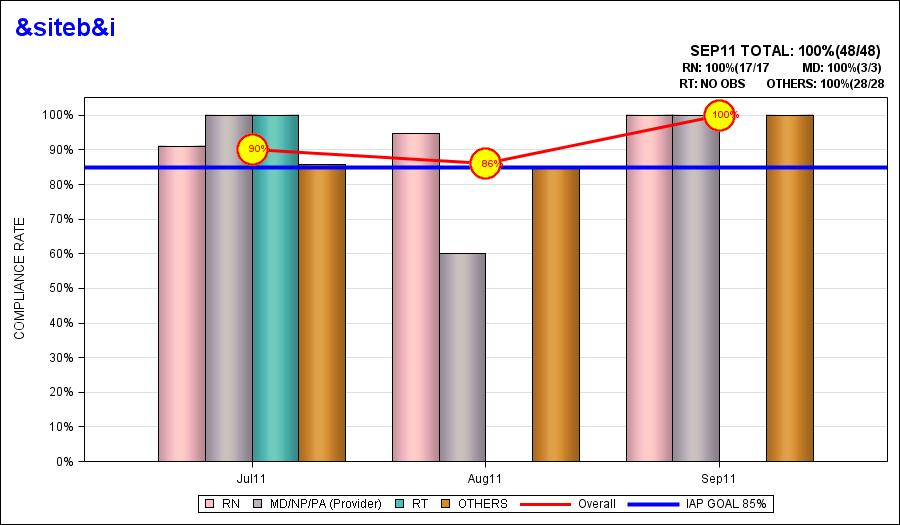## PROC GTL-eliminate missing (bars,lines,etc) from graphs-

To all,

I have a bar graph that in some months is missing one component or another that leaves an unsightly gap in the graph and confuses the viewer when it occurs.

IS there anyway to simply "migrate" the bars left or right.

My template follows below in addition to a rendering of the graph.

Any help much appreciated-

Lawrenceproc template;

define statgraph BarLineMulti;

begingraph;

entrytitle halign=left  TEXTATTRS=(color=blue size=18pt) "&&siteb&i"  ;

entrytitle halign=right   TEXTATTRS=(size=11pt) "&MONTHG TOTAL: &TOTG"  ;

entrytitle halign=right   TEXTATTRS=(size=9pt) "RN: &RNG           MD: &MDG"  ;

entrytitle halign=right   TEXTATTRS=(size=9pt) "RT: &RTG   OTHERS: &OTH";

layout overlay / xaxisopts=(display=(line ticks tickvalues )) cycleattrs=true  yaxisopts=(LABEL=('COMPLIANCE RATE') offsetmin=0.0 offsetmax=0.05 griddisplay=on linearopts=(tickvaluesequence=(start=0 end=1 increment=.1))) ;

barchart x=date y=RN / skin=modern  FILLATTRS =(color=PINK) includemissinggroup=false  discreteoffset=-0.3 barwidth=0.2 name="RN" legendlabel="RN";

barchart x=date y=MD /skin=modern FILLATTRS =(color=LIPGR) includemissinggroup=false  discreteoffset=-0.1 barwidth=0.2 NAME="MD/NP/PA (Provider)" legendlabel="MD/NP/PA (Provider)";

barchart x=date y=RT/skin=modern FILLATTRS =(color=BIBG) includemissinggroup=false discreteoffset= 0.1 barwidth=0.2 name="RT" legendlabel="RT";

barchart x=date y=OTHER /skin=modern FILLATTRS =(color=BIO) includemissinggroup=false discreteoffset= 0.3 barwidth=0.2 name="OTHERS" legendlabel="OTHERS";

seriesplot x=date y=p / lineattrs=graphdatadefault(thickness=3 color=red) name="Overall"    LEGENDLABEL="Overall" ;

ReferenceLine y=.85 / clip=true Lineattrs=( Pattern=solid thickness=4 color=blue) name="IAP GOAL 85%" legendlabel="IAP GOAL 85%";

scatterplot x=date y=P /    markerattrs=(symbol=circlefilled size=30 color=yellow) /*name="Overall"  LEGENDLABEL="Overall"*/;

scatterplot x=date y=P / markerattrs=(symbol=circle size=31 color=red) ;

scatterplot x=date y=P / markercharacter=p /*discreteoffset= -0.1*/ markercharacterattrs=(color=red weight=bold);

discretelegend   "RN" "MD/NP/PA (Provider)" "RT" "OTHERS" "Overall" "IAP GOAL 85%" /

location=outside  valign=bottom;

endlayout;

endgraph;

end;

run;

1 ACCEPTED SOLUTION

Accepted SolutionsJay54
Meteorite | Level 14

## Re: PROC GTL-eliminate missing (bars,lines,etc) from graphs-

Using SAS 9.3, to do what you want, you need to create a "grouped" data structure.  Now, instead of having a separate column for each response for RN, MD, RT, etc, you need a column for response (compliance) with a group variable (say- type) which will have the values RN, MD, etc.  Now, use one BARCHART statement:

`BARCHART x=date y=compliance / group=type groupdisplay=cluster; `
`Since the SERIESPLOT is not grouped, that data will need to be in a separate column, with only one data value for each date value..   `
`If you are using skins, change that to DATASKIN=PRESSED.`
`Feel free to email me if you have questions.`
4 REPLIES 4Jay54
Meteorite | Level 14

## PROC GTL-eliminate missing (bars,lines,etc) from graphs-

The short answer is "not by using some option".  Specific discrete offsets are set for each of the four bar statements.  So, each bar chart is offset by the amount you specified.  Any particular bar chart does not know if the other one has missing data for any one category.  If this is absolutely crucial, you could set up a barchart statement for each bar separately, and then set each discrete offset individually after taking into account missing values across all bar charts. But that may not be a scalable / portable solution.

While this is of no help to you now, the behavior you want (compressing out of unused space of missing values) is implemented in V9.3 for grouped bar charts with GROUPDISPLAY=CLUSTER.  We can do this in this case since this is one bar chart statement with groups, so it knows about the missing values, and can compress them out.

## PROC GTL-eliminate missing (bars,lines,etc) from graphs-

Sanjay, I will keep that in mind in the future as to GROUPDISPLAY=CLUSTER.

In the interim, I'll have to live with it. The concept of setting each discrete offset seems cumbersome.

I'll see whether UCSF is update to version 9.3 yet. Hope you are enjoying SF.

Lawrence

## PROC GTL-eliminate missing (bars,lines,etc) from graphs-

Sanjay,

good news-I am getting SAS 9.3. SO where exactly do I place this statement!

LawrenceJay54
Meteorite | Level 14

## Re: PROC GTL-eliminate missing (bars,lines,etc) from graphs-

Using SAS 9.3, to do what you want, you need to create a "grouped" data structure.  Now, instead of having a separate column for each response for RN, MD, RT, etc, you need a column for response (compliance) with a group variable (say- type) which will have the values RN, MD, etc.  Now, use one BARCHART statement:

`BARCHART x=date y=compliance / group=type groupdisplay=cluster; `
`Since the SERIESPLOT is not grouped, that data will need to be in a separate column, with only one data value for each date value..   `
`If you are using skins, change that to DATASKIN=PRESSED.`
`Feel free to email me if you have questions.`
Discussion stats
• 4 replies
• 1161 views
• 3 likes
• 2 in conversation